# Intro

This is an exploratory data analysis of iterated prisoner’s dilemma tournament results generated by the axelrod library. If you are unfamiliar with either, take a look at this recent blog post by Martin Jones about how he created the current best strategy in the library.

The iterated prisoner’s dilemma (IPD) is a model in game theory that is generally applied to the evolution of cooperation and to strategic interactions in many fields. The axelrod library, at the time of writing, contains approximately 100 strategies for the IPD, including most classic strategies like Tit-For-Tat (TFT), recent variants and past tournament winners like Omega-TFT, and several new strategies. Many of the strategies are parameterized and the library can generate infinitely many variations. For this analysis, I have taken the base ~100 strategies, played out 1000 matchups of 200 rounds for each pair, and summarized the data into two tables. Table_1 contains various properties derived from heads-up action between every pair of opponents, including the cooperation percentage, whether the strategies are stochastic, how many rounds of history they utilize, how often the strategies cooperate in various contexts, and more. These properties are averaged over 1000 matches of 200 rounds. Table 2 contains the data aggregated over opponents, and also includes statistical results from 100 round-robin tournaments of all the strategies.

The data was generated by a python script available in this repository and we will analyze the data using R.

# Exploratory Data Analysis

Generally we are concerned with how strategies perform heads up and in tournament play.

## Tournament Scores and Wins

First we load the data into R:

``library(dplyr)``
``````library(ggplot2)
library(gridExtra)

color_palette_function <- colorRampPalette(
colors = c("red", "orange", "blue"),
space = "Lab" # Option used when colors do not represent a quantitative scale
)

Here are the distributions for mean scores and wins in the tournaments: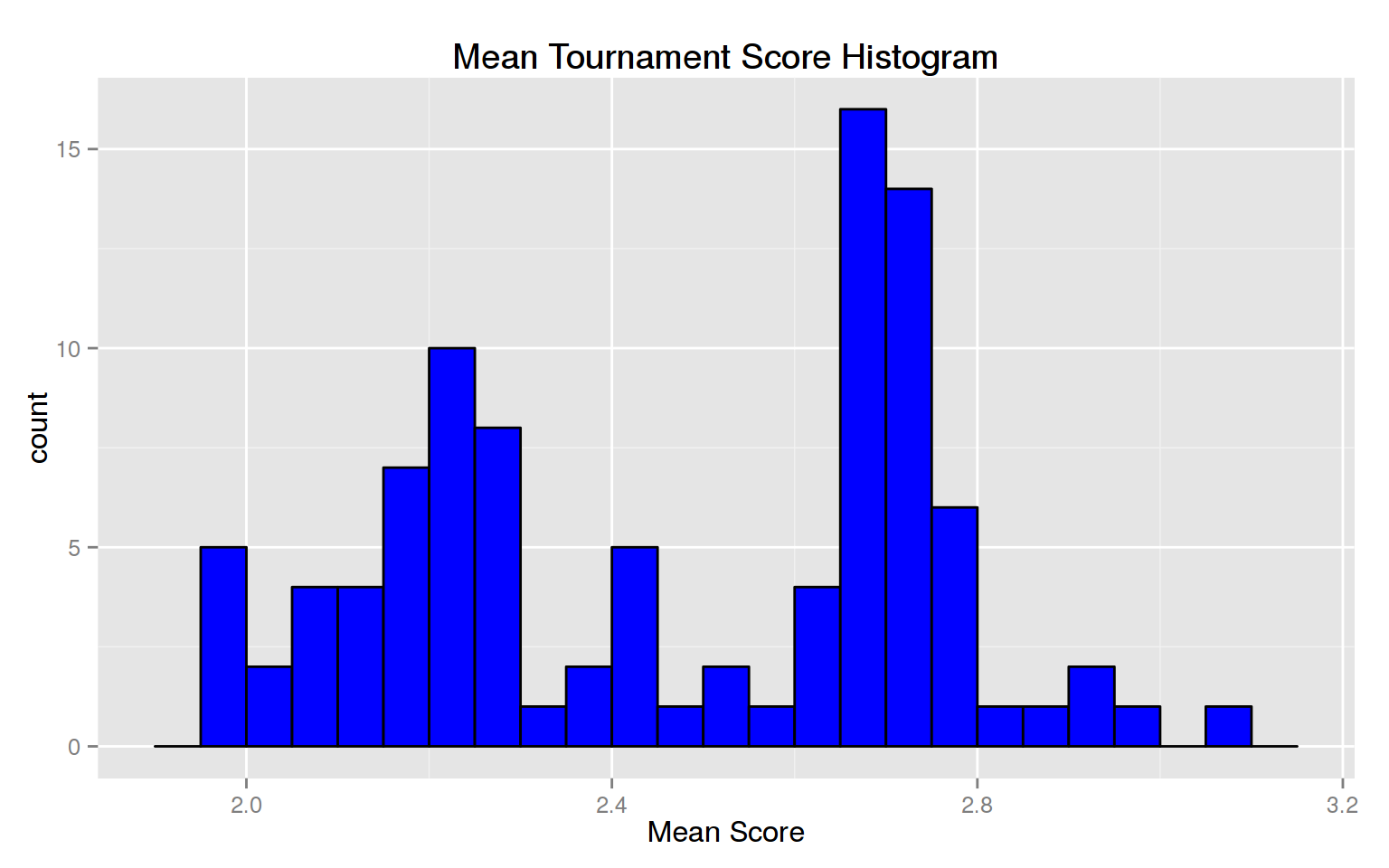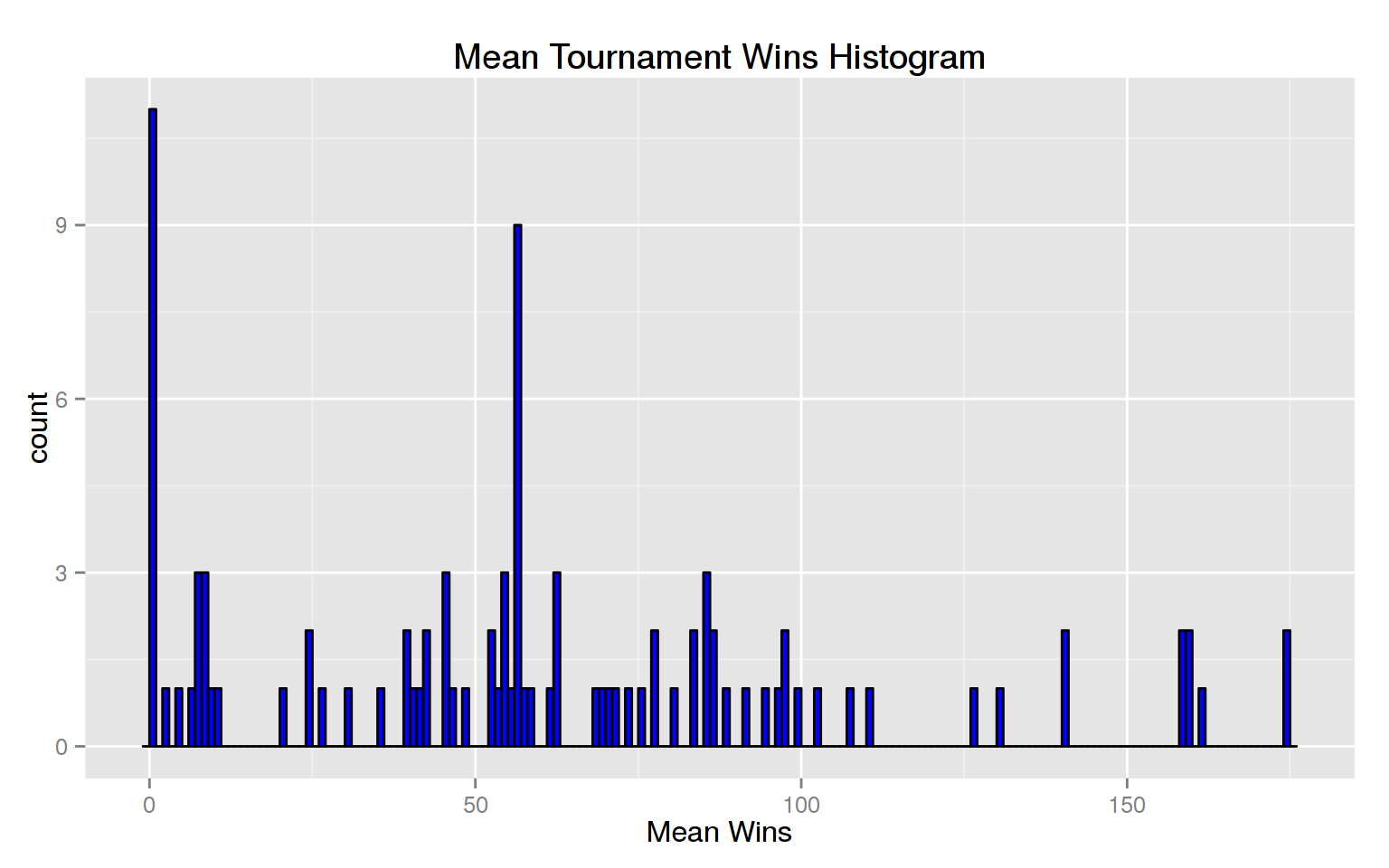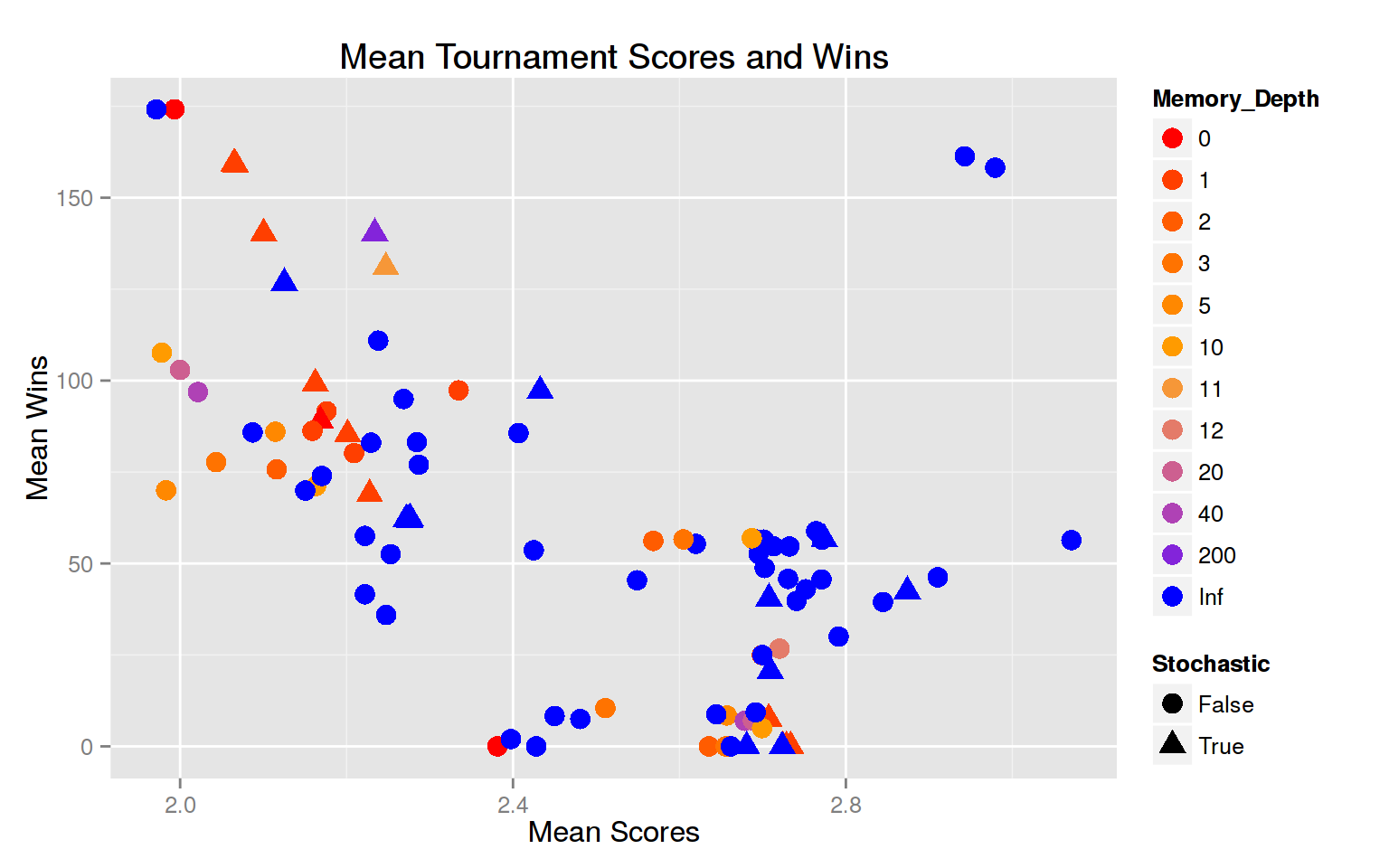Alread we notice that there appears to be a strong connection between mean score and mean wins in a given tournament, with two interesting outliers. These strategies use knowledge of the tournament length to defect on the last few moves, which is not always allowed. (We’ll toss these strategies later when we do some analysis.)

For the curious, Table 1 gives a lot of information on how each strategy fares against each other strategy. For example, for EvolvedLookerUp, we can look at the distributions of mean score per opponent (averaged over 1000 matches), and a number of other characteristics. EvolvedLookerUp manages to cooperate with many opponents (the spike at 3), exploit some opponents (scores near 5), and mutually defect against others (scores near 1). First defections are set to 200 (the end of the match) if the player never defects.

``````elu <- filter(table1, player_name == "EvolvedLookerUp")
p1 <- ggplot(elu, aes(elu\$mean_score)) + geom_histogram(fill="blue", binwidth=0.1) +
labs(x="Mean Scores", title="EvolvedLookerUp Scores")
p2 <- ggplot(elu, aes(elu\$mean_first_defection)) + geom_histogram(fill="blue", binwidth=5) +
labs(x="Mean First Defection", title="EvolvedLookerUp First Defections")
grid.arrange(p1, p2, ncol=2)``````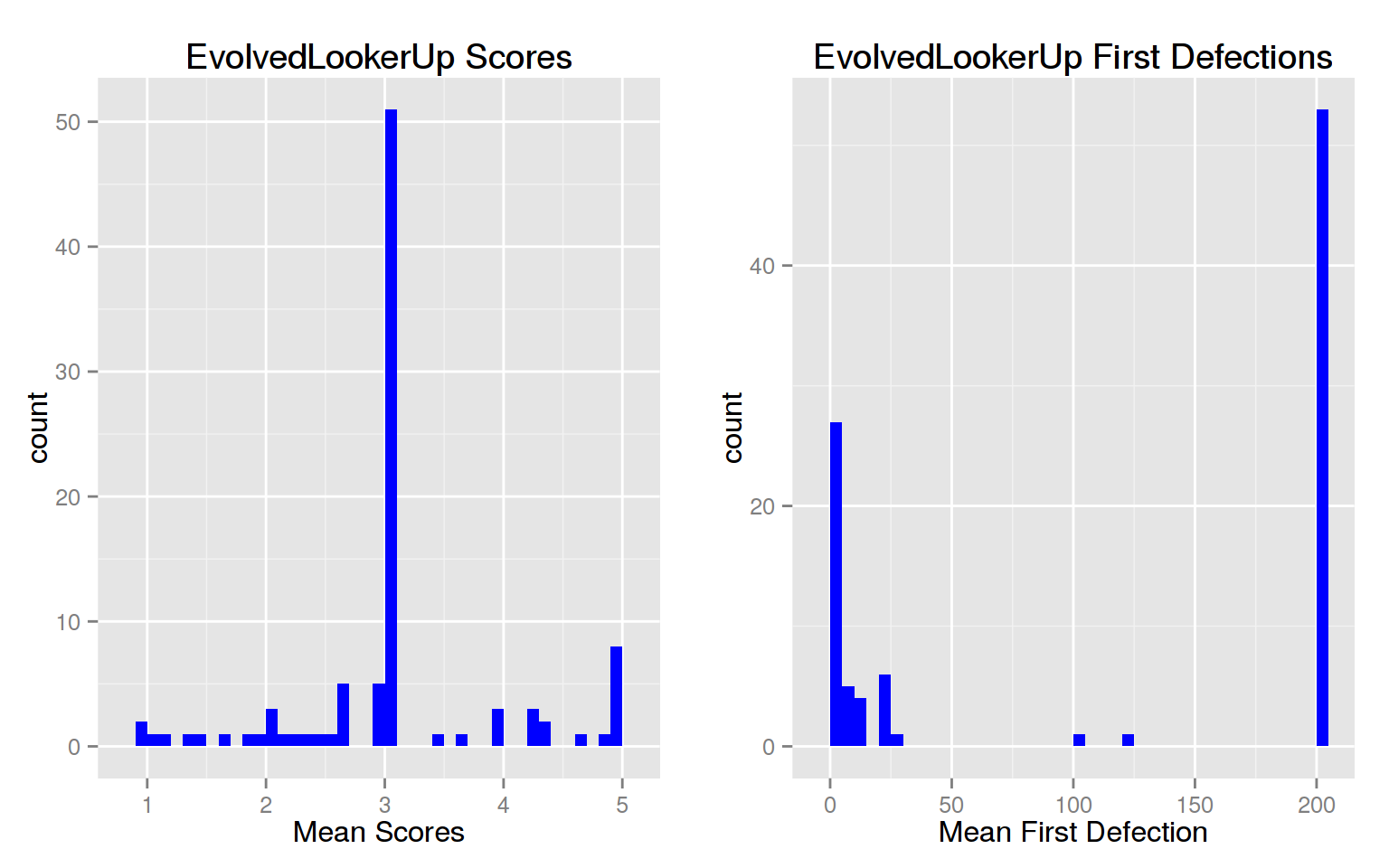## Memory Depth and Stochasticity

Every strategy in the axelrod library has a number of classifiers, including `memory_depth`, which is how many rounds of previous history a strategy uses to determine the next action, and whether or not the strategy is `stochastic`. Let’s see if either property affects performance.

``````ggplot(table2, aes(factor(memory_depth), tournament_score_mean)) +
geom_boxplot(aes(fill=factor(memory_depth))) +
ggtitle("Mean Tournament Score versus Memory Depth") +
labs(x="Memory Depth", y="Mean Score", color="Memory_Depth") +
theme(legend.title=element_blank())``````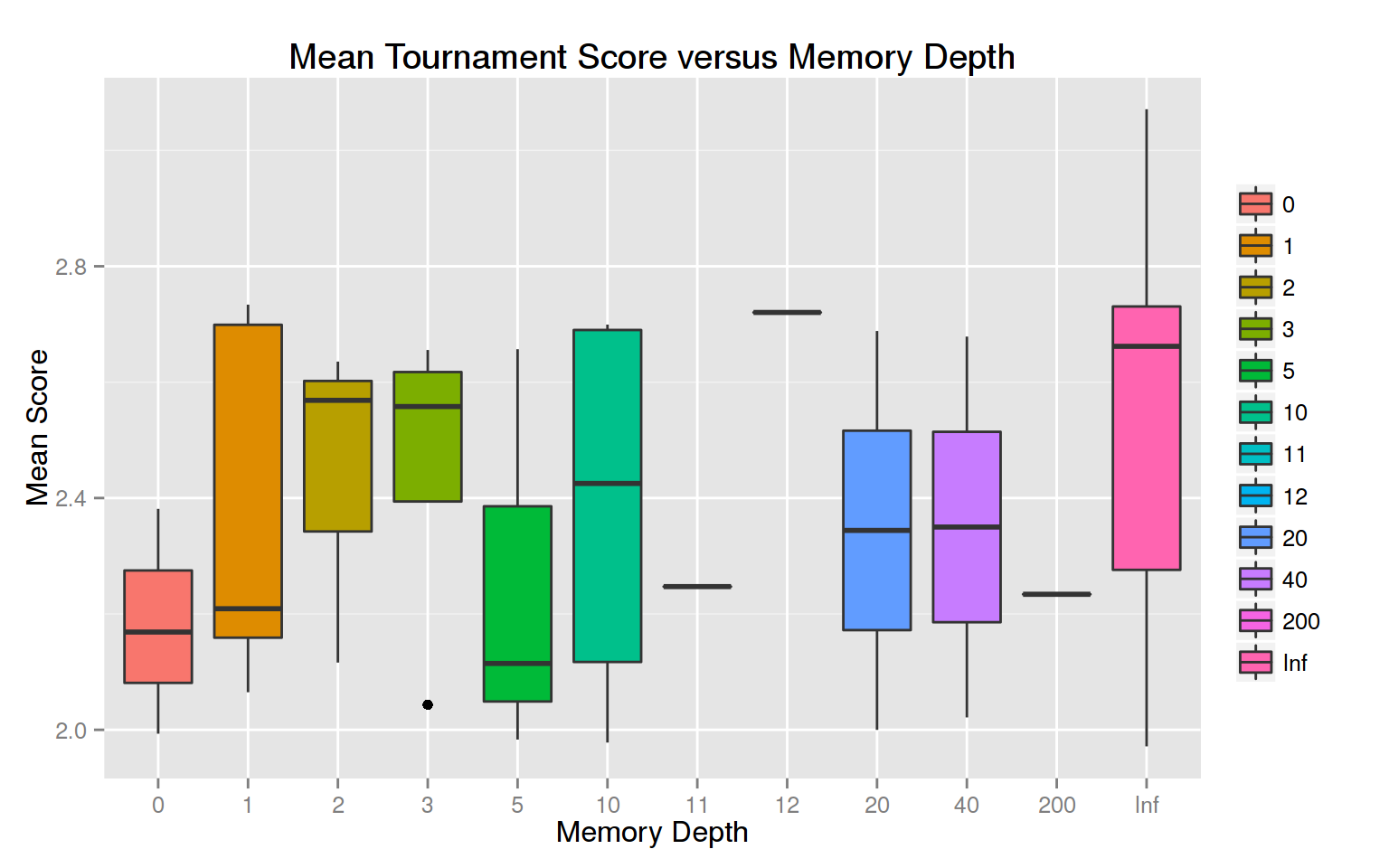``````ggplot(table2, aes(factor(memory_depth), tournament_win_mean)) +
geom_boxplot(aes(fill=factor(memory_depth))) +
ggtitle("Mean Tournament Wins versus Memory Depth") +
labs(x="Memory Depth", y="Mean Wins") +
theme(legend.title=element_blank())``````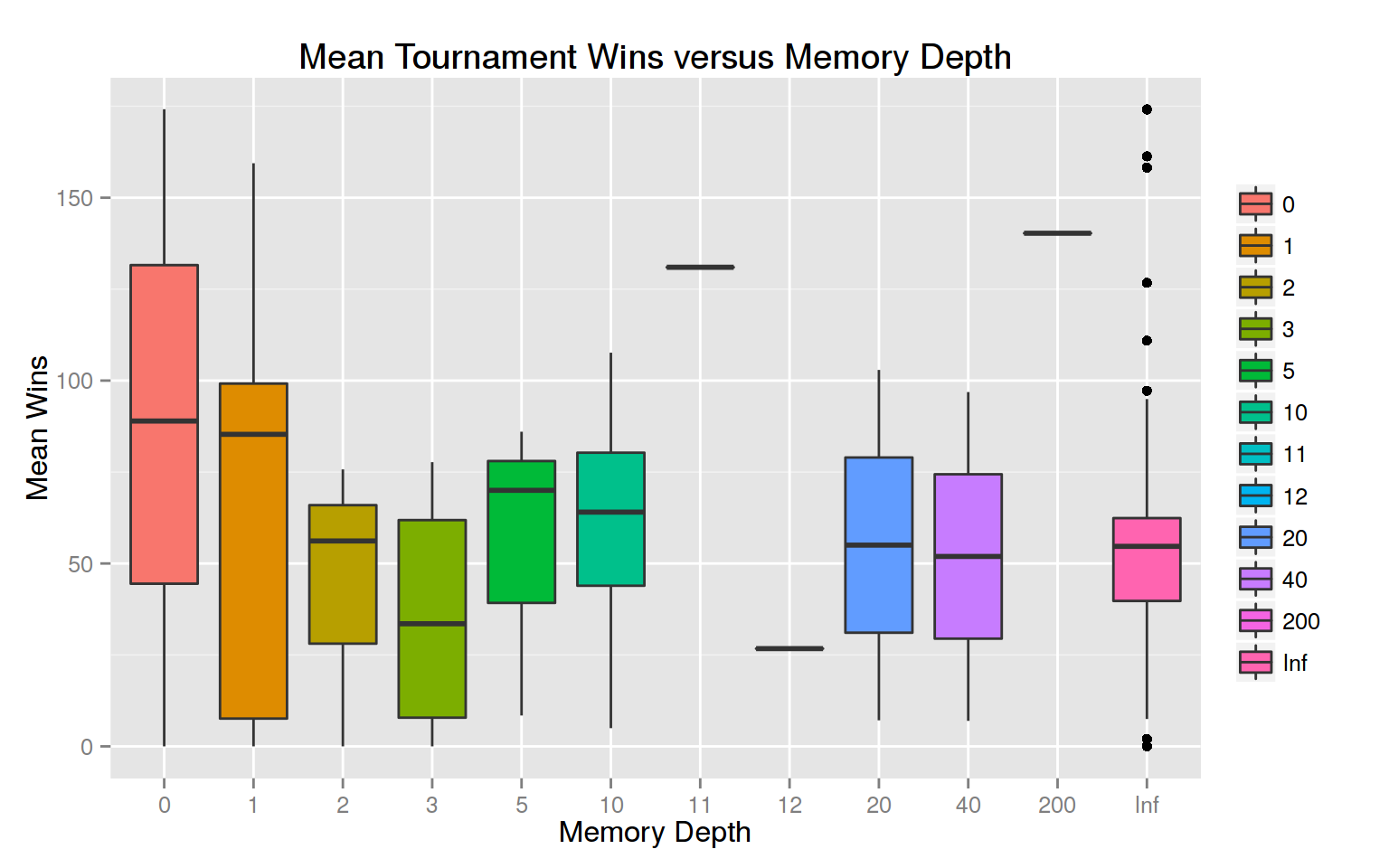Greater memory_depth does appear to correlate with mean tournament score. Some authors have claimed that in various contexts that strategies that use more than one round of memory are superfluous; clearly that is not the case here.

Being stochastic appears to be a disadvantage for tournament score, and possibly an advantage for tournament mean.

``````p1 <- ggplot(table2, aes(factor(stochastic), tournament_score_mean)) +
geom_violin(aes(fill=factor(stochastic))) +
ggtitle("Mean Tournament Scores") +
labs(x="Stochastic", y="Mean Scores") +
scale_fill_discrete(name="Stochastic")

p2 <- ggplot(table2, aes(factor(stochastic), tournament_win_mean)) +
geom_violin(aes(fill=factor(stochastic))) +
ggtitle("Mean Tournament Wins") +
labs(x="Stochastic", y="Mean Wins") +
theme(legend.title=element_blank())

grid.arrange(p1, p2, ncol=2)``````